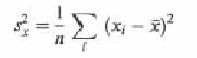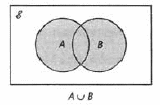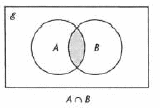Pathways To Learning... Since 2005 Hong Kong Registered School 566985

# V - value to von Koch curve - Mathematics Dictionary

A   B   C   D   E   F   G   H   I   J   K   L   M   N   O   P   Q   R   S   T   U   V   W   X   Y   Z

value: The "output" of a function. As opposed to the argument, which is the "input" of a function. This use of value can be ambiguous as value can denote the actualisation of any quantity, including the argument.

vanish: The "disappearance" of an expression as it becomes or tends to zero.

var:Symbol denoting the variance of the specified random variable.

variable: A mathematical quantity whose value can vary. As opposed to the concept of a constant. Note that the concept of a variable is subtly different from that of an unknown.

variables separable: The situation where an ordinary differential equation of 2 variables can be manipulated such as all instances of one variable (including the differential) appear on only one side of the equation and all instances of the other variable (agai including the differential) appear only on the other side, such that the equation can be integrated on both sides with respect to the corresponding variable only. It is a type of differential equations which is generally easier to solve.variance: A measure of dispersion, calculated by finding the mean of the square of numbers and the square of the mean of numbers. For a random variable X, it is denoted Var(X), calculated by finding the expected value of the square numbers subtract the square of the expected value of numbers. Only in the situation where all the numbers are the same, would the two quantities be equal, in which case the variance is zero, "no dispersion whatsoever".

variate: The value of a random variable.

variation, coefficient of: A measure of dispersion that aims to discount the effect of the size of the numbers measured in question. It is calculated byvariations in sign: It is, when a polynomial in one variable and real coefficients is written such that terms are arranged in descending order of degrees, and ignoring terms with coefficient of zero (terms which vanished), the (number of) times the parity of the coefficient switches (between positive and negative). It is used in Descartes's rule of signs to establish some bounds on the number of roots of the polynomial.

vector: A mathematical object consisting of magnitude and direction. Specifically, two vectors are considered the same as long as the aforementioned two quantities are, regardless of whether they have different "origins", "destinations" or "routes". Addition, subtraction and "multiplication" is defined for vectors. There is no division and there is a second type of "multiplication" for three dimensional vectors. Vectors are often visualised as arrows, with direction as well as length (of the tail), although the idea of vectors can generalise and abstractise beyond mere geometrical constructions.

Vectors can be denoted as an object on its own v, via two points (top arrow)AB, or through matrices (given a basis). There is a parallel convention to denote vectors in two or three dimensions through unit vectors (basis): i (positive x direction/east), j (positive y direction/north) and k (positive z direction/up).

vector product: An vector operation in three-dimensional Euclidean space. It takes two vectors and produces a third which is perpendicular to the two. The magnitude and direction of the resulting vector is determined by the two vectors in a pre-defined manner. Though distinct from multiplication for numbers, they shares enough properties for the vector product to be considered a "multiplication". e.g. distribution over addition. Although vector product is not commutative nor associative, unlike multiplication for numbers. It is commonly called the cross product due to the notation we use to distinguish it from the scalar product.

vector quantity: A quantity with magnitude as well as direction. As opposed to scalar quantities which has magnitude but not directions. Examples of vector quantities are velocity and displacement, whose scalar equivalent are speed and distance respectively. Note that acceleration as a vector quantity is different from acceleration as a scalar quantity, even though we do not commonly have distinct words for the two concepts.

vector space: A collection (set) of objects, together with a set of numbers (field), satisfying the following properties:

(1) There is an operation called addition, applied to any two elements of the vector space, that is commutative and associative.

(2) There is an operation called (scalar) multiplication, applied to a scalar (from the field) and a scalar (from the set), that is associative and distributive.

(3) There exist a unique (vector) identity for addition and a unique (scalar) identity for multiplication

(4) There exist an additive inverse for all vector elements in the set.

(4) Scalar multiplication is distributive over vector addition as well as scalar addition.

velocity: The rate of change of displacement with respect to time. Often represented by the symbol v. It is the vector quantity equivalent of speed. Intuitively, it is the quantity speed with the direction of motion taken into account.

Venn diagram: A diagram using spatial relationships (points"within" shape corresponds to an element "is a member of" a set and derived relationships such as, shape "contained in" another shape corresponds to a set "is a subset of" another set) to represent and visually represent all the combinations that an object can have regarding membership of sets featured in the diagram. Note that the sets can be represented by shapes other than circles and ovals and is sometimes required to take on other shapes in order to accurately represent the sets in question.vertex: A point in a geometric object where two or more lines meet. It is also used for a local extremum of a curve. The plural of vertex is vertices.

vigesimal numbers: Numbers expressed in the base 20 number system.

volt: The SI unit for electrical potential differences. It is equivalent to the difference in electrical potential between two points on a conductor carrying 1 ampere of current with 1 watt of power dissipated "in between". Often denoted with the symbol V.volume: A measure indicating the magnitude of space within a three dimensional object. The same concept within a two dimensional object would then be called area.

von Koch curve: A fractal curve defined as the limit of iterations where, beginning with an equilateral triangle and, at each subsequent stage, the middle third of any line is removed and replaced with two identical lines of the same length as the one removed so as to form a closed curve with a "new triangle" "pointing outwards", i.e., in such a way that the area of the closed curve increases.

A   B   C   D   E   F   G   H   I   J   K   L   M   N   O   P   Q   R   S   T   U   V   W   X   Y   Z

Genius is one percent inspiration and ninety-nine percent perspiration.